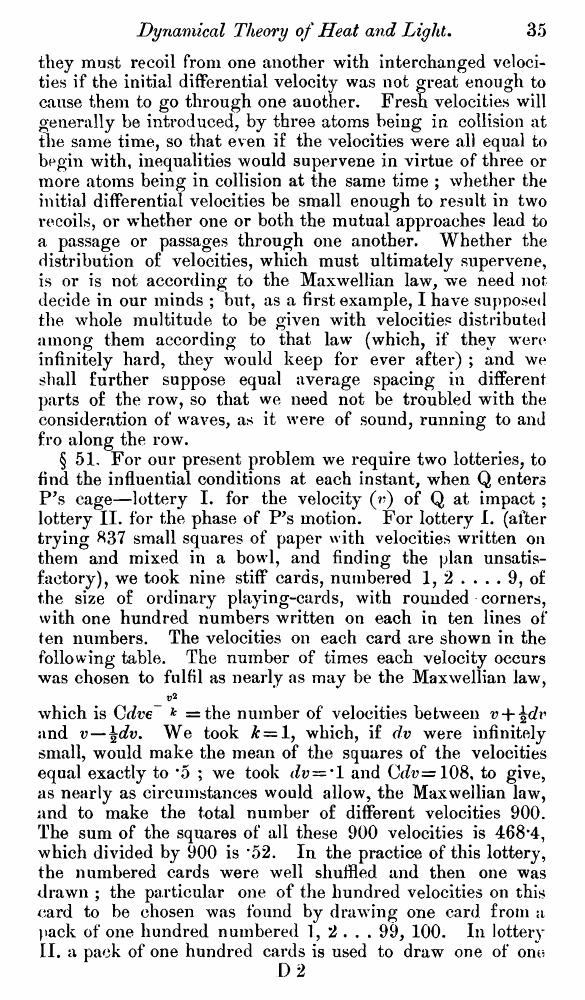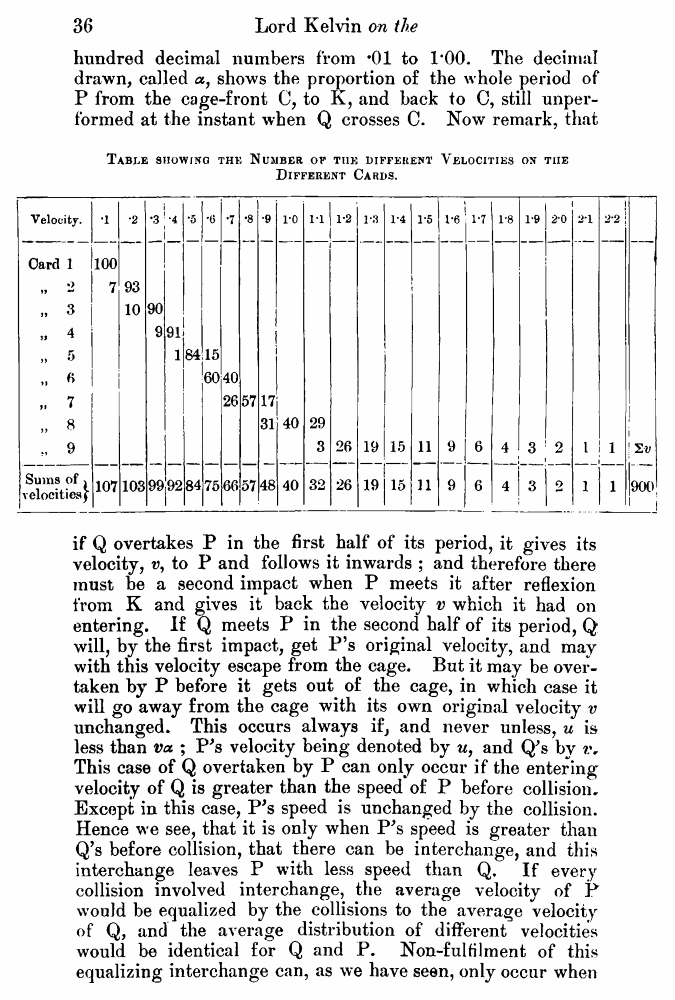﻿ ﻿ Lord Kelvin. Nineteenth-Century Clouds over the Dynamical Theory of Heat and Light. // Phil. Mag. S. 6. Vol. 2. No. 7. July 1901.

# Lord Kelvin. Nineteenth-Century Clouds over the Dynamical Theory of Heat and Light. // Phil. Mag. S. 6. Vol. 2. No. 7. July 1901.

В начало   Другие форматы   <<<     Страница 35   >>>1  2  3  4  5  6  7  8  9  10  11  12  13  14  15  16  17  18  19  20  21  22  23  24  25  26  27  28  29  30  31  32  33  34  35 36  37  38  39  40 they must recoil from one another with interchanged velocities if the initial differential velocity was not great enough to cause them to go through one auother. Fresh velocities will generally be introduced, by three atoms being in collision at the same time, so that even if the velocities were all equal to begin with, inequalities would supervene in virtue of three or more atoms being in collision at the same time ; whether the initial differential velocities be small enough to result in two recoils, or w’hether one or both the mutual approaches lead to a passage or passages through one another. Whether the distribution of velocities, which must ultimately supervene, is or is not according to the Maxwellian law, we need not decide in our minds ; but, as a first example, I have supposed the whole multitude to be given with velocities distributed among them according to that law (which, if they were infinitely hard, they would keep for ever after) ; and we shall further suppose equal average spacing in different parts of the row, so that we need not be troubled with the consideration of waves, as it were of sound, running to and fro along the row. § 51, For our present problem we require two lotteries, to find the influential conditions at each instant, when Q enters P’s cage—lottery I. for the velocity (r) of Q at impact ; lottery II. for the phase of P’s motion. For lottery I. (after trying 837 small squares of paper with velocities written on them and mixed in a bowl, and finding the plan unsatisfactory), we took nine stiff cards, numbered 1, 2 .... 9, of the size of ordinary playing-cards, with rounded corners, with one hundred numbers written on each in ten lines of* ten numbers. The velocities on each card are shown in the following table. The number of times each velocity occurs was chosen to fulfil as nearly as may be the Maxwellian law, which is Cdve~ k =the number of velocities between v + and 17—\dv. We took £ = 1, which, if dv were infinitely small, would make the mean of the squares of the velocities equal exactly to *5 ; we took dv = ' 1 and Crfv=108, to give, as nearly as circumstances would allow, the Maxwellian law, and to make the total number of different velocities 900. The sum of the squares of all these 900 velocities is 468*4, which divided by 900 is *52. In the practice of this lottery, the numbered cards were well shuffled and then one was drawn ; the particular one of the hundred velocities on this card to be chosen was found by drawing one card from a pack of one hundred numbered 1, 2 . . . 99, 100. In lottery II. a pack of one hundred cards is used to draw one of onr? D 2 hundred decimal numbers from *01 to 1*00. The decimal drawn, called a, shows the proportion of the whole period of P from the cage-front C, to K, and back to C, still unperformed at the instant when Q crosses C. Now remark, that Table showing the Number op the different Velocities on tiie Different Cards. if Q overtakes P in the first half of its period, it gives its velocity, v, to P and follows it inwards ; and therefore there must be a second impact when P meets it after reflexion from K and gives it back the velocity v which it had on entering. If Q meets P in the second half of its period, Q will, by the first impact, get P’s original velocity, and may with this velocity escape from the cage. But it may be overtaken by P before it gets out of the cage, in which case it will go away from the cage with its own original velocity v unchanged. This occurs always if, and never unless, u is less than va ; P's velocity being denoted by u, and Q's by This case of Q overtaken by P can only occur if the entering velocity of Q is greater than the speed of P before collision. Except in this case, P's speed is unchanged by the collision. Hence we see, that it is only when P's speed is greater than Q’s before collision, that there can be interchange, and this interchange leaves P with less speed than Q. If every collision involved interchange, the average velocity of P would be equalized by the collisions to the average velocity of Q, and the average distribution of different velocities would be identical for Q and P. Non-fulfilment of this equalizing interchange can, as we have seen, only occur when